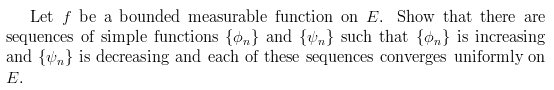# Showing that there are particular sequences of functions that convergeThat seems like a valid argument for showing that $\phi_n$ converges to f, but I'm not sure how to show it's increasing. And as far $\psi_n$, converges, well I imagine that I'd use a similar argument, but I'm still not sure how to show it's decreasing.

Since $\phi_n$ is smaller than f, you can define
$$\tilde{\phi_n}(x) = \max_{j=1,...,n} \phi_k(x)$$
and this will still be within 1/n of f everywhere, and is increasing in n, and each $\tilde{\phi_n}$ is a simple function. Exercise to prove all these properties.